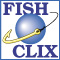Welcome to FishClix.com
Webmaster's Marketing Tools For The Angling Site

• " . (\$link['BeforeText'] ? \$link['BeforeText'] . ' ' : '') . '' . \$link['Text'] . '' . (\$link['AfterText'] ? ' ' . \$link['AfterText'] : '') . "
• \n"; } echo ''; } } function tla_updateLocal(\$url, \$file, \$time_out) { touch(\$file); if (\$xml = file_get_contents_tla(\$url, \$time_out)) { if (\$handle = fopen(\$file, 'w')) { fwrite(\$handle, \$xml); fclose(\$handle); } } } function tla_getLocal(\$file) { if (function_exists('file_get_contents')) { return file_get_contents(\$file); } \$contents = ''; if (\$handle = fopen(\$file, 'r')) { \$contents = fread(\$handle, filesize(\$file) + 1); fclose(\$handle); } return \$contents; } function file_get_contents_tla(\$url, \$time_out) { \$result = ''; \$urlInfo = parse_url(\$url); if (\$handle = @fsockopen(\$urlInfo['host'], 80)) { if (function_exists('socket_set_timeout')) { socket_set_timeout(\$handle, \$time_out, 0); } else if (function_exists('stream_set_timeout')) { stream_set_timeout(\$handle, \$time_out, 0); } fwrite(\$handle, 'GET ' . \$urlInfo['path'] . '?' . \$urlInfo['query'] . " HTTP/1.0\r\nHost: " . \$urlInfo['host'] . "\r\nConnection: Close\r\n\r\n"); while (!feof(\$handle)) { \$result .= @fread(\$handle, 40960); } fclose(\$handle); } else if (function_exists('curl_init')) { \$ch = curl_init(); curl_setopt(\$ch, CURLOPT_URL, \$url); curl_setopt(\$ch, CURLOPT_RETURNTRANSFER, 1); curl_setopt(\$ch, CURLOPT_CONNECTTIMEOUT, \$time_out); curl_setopt(\$ch, CURLOPT_TIMEOUT, \$time_out); curl_setopt(\$ch, CURLOPT_FOLLOWLOCATION, 1); \$result = curl_exec(\$ch); curl_close(\$ch); } \$return = ''; \$capture = false; foreach (explode("\n", \$result) as \$line) { \$char = substr(trim(\$line), 0, 1); if (\$char == '[' || \$char == '<') { \$capture = true; } if (\$capture) { \$return .= \$line . "\n"; } } return \$return; } function tla_decode(\$str) { if (!function_exists('html_entity_decode')) { function html_entity_decode(\$string) { // replace numeric entities \$string = preg_replace('~&#x([0-9a-f]+);~ei', 'chr(hexdec("\1"))', \$string); \$string = preg_replace('~&#([0-9]+);~e', 'chr(\1)', \$string); // replace literal entities \$trans_tbl = get_html_translation_table(HTML_ENTITIES); \$trans_tbl = array_flip(\$trans_tbl); return strtr(\$string, \$trans_tbl); } } if (substr(\$str, 0, 1) == '[') { \$arr = json_decode(\$str, true); foreach (\$arr as \$i => \$a) { foreach (\$a as \$k => \$v) { \$arr[\$i][\$k] = tla_decode_str(\$v); } } return \$arr; } \$out = ''; \$retarr = ''; preg_match_all("/<(.*?)>(.*?) \$retarr['BeforeText'][\$i], 'URL' => \$retarr['URL'][\$i], 'Text' => \$retarr['Text'][\$i], 'AfterText' => \$retarr['AfterText'][\$i], ); } return \$arr; } function tla_decode_str(\$str) { \$search_ar = array('<', '>', '"'); \$replace_ar = array('<', '>', '"'); return str_replace(\$search_ar, \$replace_ar, html_entity_decode(strip_tags(\$str))); } tla_ads(); ?>